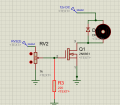# Is it possible to control the Output of a motor using PWM ?

Joined May 11, 2018
126
Hello i am trying to create a PID system with arduino and motor and im trying to varry the motor speed as the PV reaches the setpoint....

So the power comes from the arduino (replaced the arduino by a POT for demonstration purposes )and goes to the gate of a MOSFET , but i thaught the MOSFET job is just to switch on and OFF which means that in my point of view the speed of the motor will always be the same as long as the gate is switched on whether its voltage is varying or not .( but i dont know i may be wrong as well). since to me the motor is connected to 12V..

So my question goes like this ,if you change the voltage at the gate with PWM will it change the spped of the motor ?

Thank you very much ....#### jpanhalt

Joined Jan 18, 2008
9,755
So my question goes like this ,if you change the voltage at the gate with PWM will it change the spped of the motor ?

Thank you very much ....
Your potentiometer is not providing PWM. It is providing a fixed voltage. With respect to that, whether changing the pot will change motor speed, that depends. Above a certain voltage the mosfet will be "fully on" and increasing that voltage will have little or no effect.

If you operate above Vth and below the fully on voltage then you are in a "linear" region and changing the voltage will affect the Rds of the mosfet. It will also generate a lot more heat (W=I^2R).

Look at the datasheet. There will be a graph of Vgs and current, voltage drop or some other measure of Rds.

#### Irving

Joined Jan 30, 2016
814
Yes it will. but you are not doing PWM with that circuit.

If you were, The motor speed is given by Kv . V where Kv is the motor constant and V the applied voltage. When you apply a pulsed voltage to the motor, it's mechanical inertia integrates to give an average speed based on the on/off ratio, assuming that is fast enough (typically around 500Hz) . The average or RMS voltage seen by the motor is given by Vrms = V . √D where D is the duty cycle (on/off ratio)

Last edited:

Joined May 11, 2018
126
Your potentiometer is not providing PWM. It is providing a fixed voltage. With respect to that, whether changing the pot will change motor speed, that depends. Above a certain voltage the mosfet will be "fully on" and increasing that voltage will have little or no effect.

If you operate above Vth and below the fully on voltage then you are in a "linear" region and changing the voltage will affect the Rds of the mosfet. It will also generate a lot more heat (W=I^2R).

Look at the datasheet. There will be a graph of Vgs and current, voltage drop or some other measure of Rds.
Hello is the drain source resistance , the same as a LOAD ?

#### jpanhalt

Joined Jan 18, 2008
9,755
Hello is the drain source resistance , the same as a LOAD ?
No. The load is what's attached between the drain and Vcc.

Joined May 11, 2018
126
No. The load is what's attached between the drain and Vcc.
Aaah ok so what is the role of an Rds in this case ?

#### Irving

Joined Jan 30, 2016
814
Hello is the drain source resistance , the same as a LOAD ?
No, Rds (drain-source resistance) is a measure of how 'open' the channel is and is related to gate voltage over a very tiny portion of the Vgs-Ids curve. The LOAD would be the effective impedance of whatever the drain-source current flows through. In the OP example its the motor windings. Effective impedance is not just the series element but any parallel element from drain to ground that absorbs energy from the circuit.

Rds is important as it determines one (static) component of the power dissipated in the MOSFET given by P = Ids . Rds ^ 2

#### jpanhalt

Joined Jan 18, 2008
9,755
Rds is the resistance of the mosfet when Vgs is equal to or greater than Vth.